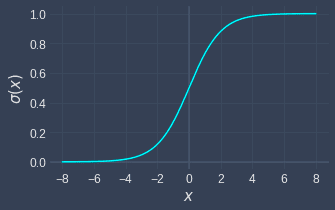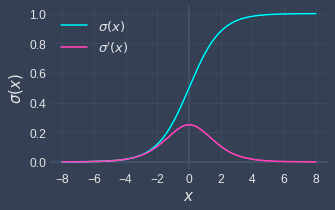search
Search
Guest 0reps
Thanks for the thanks!
close
chevron_left Activation functions
Cancel
Post
account_circle
Profile
exit_to_app
Sign out
search
keyboard_voice
close
Searching Tips
Search for a recipe:
"Creating a table in MySQL"
Search for an API documentation: "@append"
Search for code: "!dataframe"
Apply a tag filter: "#python"
Useful Shortcuts
/ to open search panel
Esc to close search panel
to navigate between search results
d to clear all current filters
Enter to expand content previewDoc SearchCode Search BetaSORRY NOTHING FOUND!
mic
Start speaking...Voice search is only supported in Safari and Chrome.
Shrink
Navigate to
A
A
brightness_medium
share
arrow_backShareTwitterFacebook
chevron_left Activation functions
0
thumb_down
0
chat_bubble_outline
0
auto_stories new
settings

# Comprehensive Guide on Sigmoid Function

Machine Learning
chevron_right
Neural Networks
chevron_right
Activation functions
schedule Jul 17, 2022
Last updated
local_offer Machine LearningPython
Tags
Definition.

# What is the sigmoid function?

The sigmoid function, often denoted as $\sigma(x)$, is defined as follows:

$$\sigma(x)=\frac{1}{1+e^{-x}}=\frac{e^x}{1+e^x}$$

The sigmoid function is named as such because it is S-shaped, as can be seen from its graph:Note the following:

• the domain of the sigmoid function is unbounded from $-\infty$ to $\infty$.

• the range is bounded between $0$ and $1$; the output can become extremely close to $0$ or $1$, but it will never reach the boundaries.

Theorem.

# Derivative of Sigmoid Function

The beauty of the sigmoid function is that its derivative is extremely clean:

$$\sigma'(x)=\sigma(x)\cdot\left[1-\sigma(x)\right]$$

This is a very useful property because we often encounter situations in machine learning where we need to compute the derivative of the sigmoid function. For instance, the update rule for gradient descent in logistic regression requires this derivative.

The proof does not require any advanced calculus:

\begin{align*} \frac{d\sigma(x)}{dx}&=\frac{d}{dx}\left(\frac{1}{1+e^{-x}}\right) \\ &=\frac{d}{dx}(1+e^{-x})^{-1} \\ &=-(1+e^{-x})^{-2}\cdot \frac{d}{dx}(1+e^{-x}) \\ &=-(1+e^{-x})^{-2}\cdot (-e^{-x}) \\ &=\frac{e^{-x}}{(1+e^{-x})^2} \\ &=\frac{1}{1+e^{-x}}\left(\frac{e^{-x}}{1+e^{-x}}\right) \\ &=\frac{1}{1+e^{-x}}\left(\frac{1+e^{-x}-1}{1+e^{-x}}\right) \\ &=\frac{1}{1+e^{-x}}\left(\frac{1+e^{-x}}{1+e^{-x}}-\frac{1}{1+e^{-x}}\right) \\ &=\frac{1}{1+e^{-x}}\left(1-\frac{1}{1+e^{-x}}\right) \\ &=\sigma(x)\cdot \left(1-\sigma (x)\right) \\ \end{align*}

## Graph of Sigmoid function and its derivative

Here's a graph of the sigmoid function and its derivative:# Implementation using Python

Here's the sigmoid function as well as its derivative implemented in Python using NumPy:

 import numpy as npdef sigmoid(x): return np.exp(x) / (1 + np.exp(x))def sigmoid_derivative(x): return sigmoid(x) * (1 - sigmoid(x)) 
mail